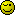# Sum values from a foreach loop

I’m using a foreach loop to write out the rows of a table which contains some numeric data from arrays amongst other things. For a couple of columns I need to sum the value of the numeric data in the variables, which ordinarily wouldn’t be a problem using a for loop, but because I’m using a foreach loop in the table, of course all the variables I’m wanting to add have the same name, \$quantity. There’s probably a ridiculously easy answer to this that I’m missing and can’t see the wood for the trees.

Could anyone give me a pointer please?

Use an array, \$quantity = first variable, \$quantity = second.
Try using this in your foreach loop.
\$x = 0; (before the foreach loop)
\$quantity[\$x] = value
\$x++;

Hope you understand what I meanSorry, I’m being a bit dense today - I put all three lines before the foreach?

No, only the \$x = 0; (so it doesn’t get redefined during the loop).

Where you normally defined \$quantity, you just replace with \$quantity[\$x]
and at the very end of the foreach loop (still inside) you put \$x++

Thanks. That makes sense now. So then I should be able to use something like:

``````\$a = array(\$quantity, \$quantity);
echo "array_sum(\$a)";
``````

to get the total?

I don’t get any errors with that, but neither do I get a total - I’m presuming it’s possibly a problem with local variables as the \$a array’s outside the foreach loop while the \$quantity is inside the loop, as when I try one of the examples given in the PHP manual:

``````\$b = array(2, 4, 6, 8);
echo "sum(b) = " . array_sum(\$b) . "\
";
``````

…that works just fine

The problem with the first block is that you used array_sum in the string.
Which just outputs array_sum.
In the second block, array_sum is out of the string and therefore parsed instead of just written on the screen.

``````
\$a = array(\$quantity, \$quantity);
echo array_sum(\$a);

``````

or

``````
\$a = array(\$quantity, \$quantity);
echo "The total is: ".array_sum(\$a);

``````
``````
\$sum = 0;
foreach(\$quantity as \$value) \$sum = \$sum + \$value;
echo \$sum;

``````

This instances the \$sum variable to 0 and then adds the value of each \$quantity to it.

Thank you so much - you’re a star! Works perfectly.Dunno if you mean me.
But if you do, npMe too then… No Problem:)

It was tbakerisageek’s suggestion I used in the end, but thanks for the help to both of you.